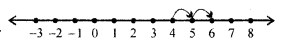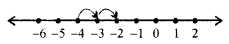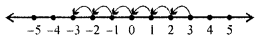## ML Aggarwal Class 6 Solutions for ICSE Maths Chapter 3 Integers Ex 3.3

Question 1.
Evaluate the following, using the number line:
(i) 4 – (-2)
(ii) -4 – (-2)
(iii) 3 – 6
(iv) -3 – (-5)
Solution:
(i) Start from 4 on the number line.
Move 2 units to the digits we reach at 6
∴ 4 – (-2) = 4 + 2 = 6(ii) Start from -4 on the number line.
Move 2 units to the right, we reach at -2
∴ -4 – (-2) = —4 + 2 = -2(iii) Start from 3 on the number line.
Move 6 units to the left, we reach at -3
3 – 6 = -3(iv) Start from -3 on the number line.
Move 5 units to the right, we reach at 2
-3 – (-5) = -3 + 5 = 2Question 2.
Subtract :
(i) -6 from 9
(ii) 6 from -9
(iii) -6 from -9
(iv) -725 from -63
(v) -376 from 10
(vi) 92 from -620
Solution:
(i) 9 – (-6) = 9 + 6 = 15
(ii) -9 – 6 = -15
(iii) -9 – (-6) = -9 + 6 = -3
(iv) -63 – (-725) = -63 + 725 = +662
(v) 10 – (-376) = 10 + 376 = 386
(vi) -620 – 92 = -712

Question 3.
Evaluate the following:
(i) -237 – (+ 1884)
(ii) -346 – (- 1275)
(iii) -190 – (-3512)
(iv) -2718 – (+ 6827)
Solution:
(i) -237 – (+ 1884)
= -237 – 1884
= -(237 + 1884) = -2121

(ii) -346 -(- 1275)
= -346 + 1275
= 1275 – 346 = 929

(iii) -190 – (-3512)
= -190 + 3512
= 3512- 190 = 3322

(iv) 2718 – (+ 6827)
= -2718 – 6827
= -(2718 + 6827) = -9545

Question 4.
The sum of two integers is 17. If one of them is -35, find the other.
Solution:
One number = -35
Sum of two integers =17
Second number = Sum of integers – (The given number)
= 17 – (-35)
= 17 + 35 = 52

Question 5.
What must be added to -23 to get -9?
Solution:
Let the number to be added = x
∴ -23 + x = -9
∴ The required number = -9 – (-23)
= -9 + 23 = 14

Question 6.
Find the predecessor of 0.
Solution:
Predecessor of 0 = 0 – 1 = -1

Question 7.
Find the successor and the predecessor of the following integers:
(i) -31
(ii) -735
(iii) -240
Solution:
(i) Successor of -31 = -31 + 1 = -30
Predecessor of -31 = -31 – 1 = -32
(ii) Successor of -735 = -735 + 1 = -734
Predecessor of-735 = -735 – 1 = -736
(iii) Successor of -240 = -240 + 1 = -239
Predecessor of -240 = -240 – 1 = -241